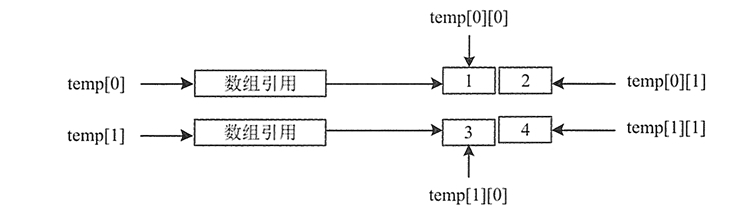# Java二维数组详解## 创建二维数组

``type arrayName[][];    // 数据类型 数组名[][];``

``type[][] arrayName;    // 数据类型[][] 数组名;``

``````int[][] age;
char[][] sex;``````

## 初始化二维数组

``````type[][] arrayName = new type[][]{值 1,值 2,值 3,…,值 n};    // 在定义时初始化
type[][] arrayName = new type[size1][size2];    // 给定空间，在赋值
type[][] arrayName = new type[size][];    // 数组第二维长度为空，可变化``````

## 例1

``int[][] temp = new int[][]{{1,2},{3,4}};````int[][] temp = new int;``

``int[][] temp = new int[];``

## 获取单个元素

``arrayName[i-1][j-1];``

``````public static void main(String[] args) {
double[][] class_score = {{10.0,99,99},{100,98,97},{100,100,99.5},{99.5,99,98.5}};
System.out.println("第二行第二列元素的值："+class_score);
System.out.println("第四行第一列元素的值："+class_score);
}``````

``````第二行第二列元素的值：98.0

## 获取全部元素

``````public static void main(String[] args) {
double[][] class_score = { { 100, 99, 99 }, { 100, 98, 97 }, { 100, 100, 99.5 }, { 99.5, 99, 98.5 } };
for (int i = 0; i < class_score.length; i++) { // 遍历行
for (int j = 0; j < class_score[i].length; j++) {
System.out.println("class_score[" + i + "][" + j + "]=" + class_score[i][j]);
}
}
}``````

``````class_score=100.0
class_score=99.0
class_score=99.0
class_score=100.0
class_score=98.0
class_score=97.0
class_score=100.0
class_score=100.0
class_score=99.5
class_score=99.5
class_score=99.0
class_score=98.5``````

``````public class Test11 {
public static void main(String[] args) {
// 创建一个二维矩阵
int[][] matrix = new int;
// 随机分配值
for (int i = 0; i < matrix.length; i++) {
for (int j = 0; j < matrix[i].length; j++) {
matrix[i][j] = (int) (Math.random() * 10);
}
}
System.out.println("下面是程序生成的矩阵\n");
// 遍历二维矩阵并输出
for (int k = 0; k < matrix.length; k++) {
for (int g = 0; g < matrix[k].length; g++) {
System.out.print(matrix[k][g] + "");
}
System.out.println();
}
}
}``````

``````下面是程序生成的矩阵

78148
69230
43823
75663
05688``````

for each循环语句不能自动处理二维数组的每一个元素。它是按照行，也就是一维数组处理的。要想访问二维教组a的所有元素，需要使用两个嵌套的循环，如下所示：

``````for (double[] row : a) {
for (double value : row) {
......
}
}``````

``````public static void main(String[] args) {
double[][] class_score = { { 100, 99, 99 }, { 100, 98, 97 }, { 100, 100, 99.5 }, { 99.5, 99, 98.5 } };
for (double[] row : class_score) {
for (double value : row) {
System.out.println(value);
}
}
}``````

``````100.0
99.0
99.0
100.0
98.0
97.0
100.0
100.0
99.5
99.5
99.0
98.5``````

``System.out.println(Arrays.deepToString(arrayName));``

``System.out.println(Arrays.deepToString(class_score));``

``[[100.0, 99.0, 99.0], [100.0, 98.0, 97.0], [100.0, 100.0, 99.5], [99.5, 99.0, 98.5]]``

## 获取整行元素

``````public static void main(String[] args) {
double[][] class_score = { { 100, 99, 99 }, { 100, 98, 97 }, { 100, 100, 99.5 }, { 99.5, 99, 98.5 } };
Scanner scan = new Scanner(System.in);
System.out.println("当前数组只有" + class_score.length + "行，您想查看第几行的元素？请输入：");
int number = scan.nextInt();
for (int j = 0; j < class_score[number - 1].length; j++) {
System.out.println("第" + number + "行的第[" + j + "]个元素的值是：" + class_score[number - 1][j]);
}
}``````

``````当前数组只有4行，您想查看第几行的元素？请输入：
3

## 获取整列元素

``````public static void main(String[] args) {
double[][] class_score = { { 100, 99, 99 }, { 100, 98, 97 }, { 100, 100, 99.5 }, { 99.5, 99, 98.5 } };
Scanner scan = new Scanner(System.in);
System.out.println("您要获取哪一列的值？请输入：");
int number = scan.nextInt();
for (int i = 0; i < class_score.length; i++) {
System.out.println("第 " + (i + 1) + " 行的第[" + number + "]个元素的值是" + class_score[i][number]);
}
}``````

``````您要获取哪一列的值？请输入：
2有用分享